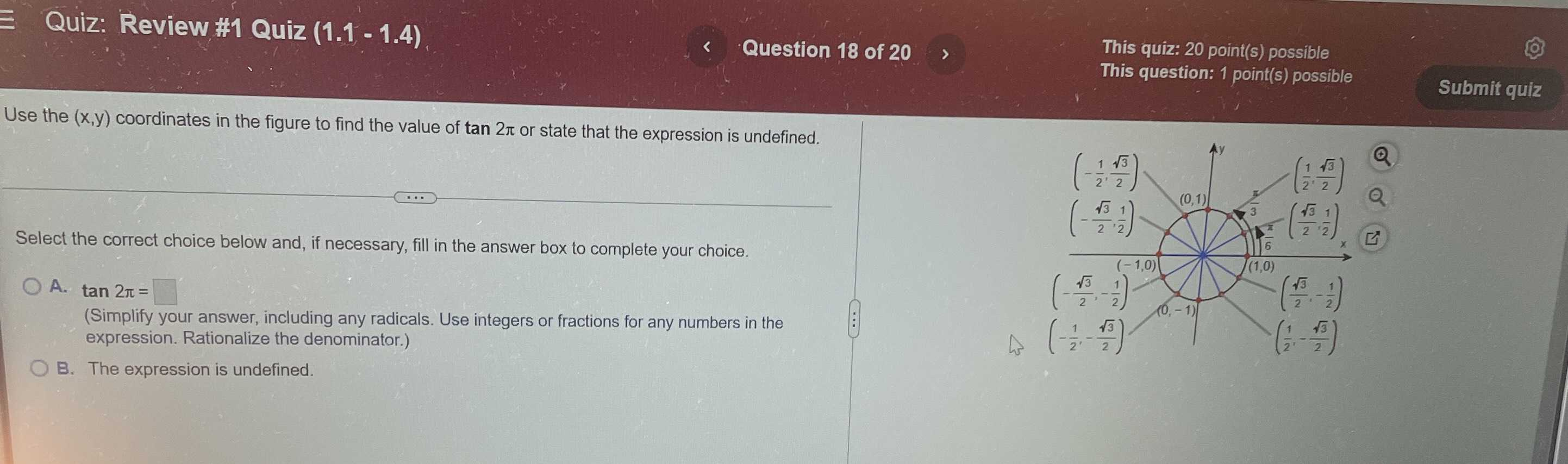### Still have math questions?

Trigonometry
QuestionUse the $$( x , y )$$ coordinates in the figure to find the value of tan $$2 \pi$$ or state that the expression is undefined. Select the correct choice below and, if necessary, fill in the answer box to complete your choice. $$18$$ of $$20,20$$ point(s) possible

A. tan $$2 \pi =$$

(Simplify your answer, including any radicals. Use integers or fractions for any numbers in the possible expression. Rationalize the denominator. )

B. The expression is undefined.